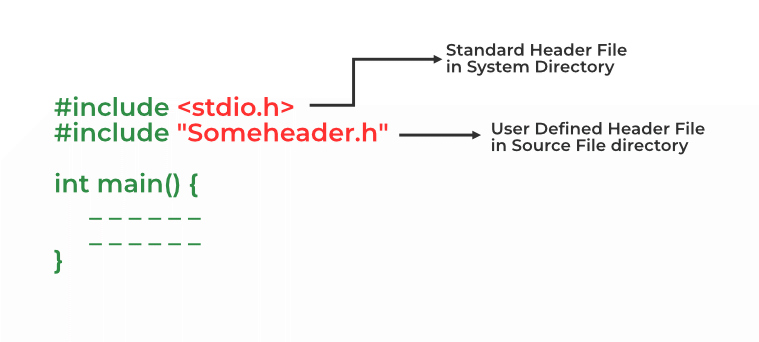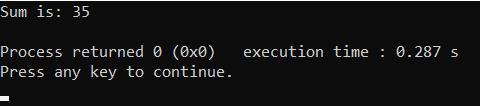# Header Files in C/C++ and its uses

In C language, header files contain a set of predefined standard library functions. The .h is the extension of the header files in C and we request to use a header file in our program by including it with the C preprocessing directive “#include”. C language has numerous libraries that include predefined functions to make programming easier.

C++ Language also offers its users a variety of functions, one of which is included in header files. In C++, all the header files may or may not end with the “.h” extension.

It offers the features like library functions, data types, macros, etc by importing them into the program with the help of a preprocessor directive “#include”. These preprocessor directives are used to instruct the compiler that these files need to be processed before compilation.

## Syntax of Header Files in C/C++

We can include header files in C by using one of the given two syntaxes whether it is a pre-defined or user-defined header file.

```#include <filename.h>    // for files in system/default directory
or
#include "filename.h"    // for files in same directory as source file```

The “#include” preprocessor directs the compiler that the header file needs to be processed before compilation and includes all the necessary data types and function definitions.## C

 `// C program to demonstrate the use of header files` `//    standard input and output stdio.h header file` `#include `   `int` `main()` `{` `    ``printf``(` `        ``"Printf() is the function in stdio.h header file"``);` `    ``return` `0;` `}`

## C++

 `// C++ program to demonstrate the use of header files` `//    standard input and output header file iostream` `#include ` `using` `namespace` `std;`   `int` `main()` `{`   `    ``cout << ``"cout is a method of iostream header file"``;` `    ``return` `0;` `}`

Output

`Printf() is the function in stdio.h header file`

There are two types of header files in C and C++:

1. Standard / Pre-existing header files
2. Non-Standard / User-defined header files

## 1. Standard Header Files in C and their Uses

Standard header files contain the libraries defined in the ISO standard of the C programming language. They are stored in the default directory of the compiler and are present in all the C compilers from any vendor.

There are 31 standard header files in the latest version of C language. Following is the list of some commonly used header files in C:

Example:

## C

 `// C program to illustrate` `// the use of header file` `// in C` `#include ` `#include ` `#include ` `#include `   `// Driver Code` `int` `main()` `{` `    ``char` `s1 = ``"12345"``;` `    ``char` `s2 = ``"Geeks"``;` `    ``char` `s3 = ``"ForGeeks"``;` `    ``long` `int` `res;`   `    ``// Find the value of 9^3 using a` `    ``// function in math.h library` `    ``res = ``pow``(9, 3);` `    ``printf``(``"Using math.h, "` `           ``"The value is: %ld\n"``,` `           ``res);`   `    ``// Convert a string to long long int` `    ``// using a function in stdlib.h library` `    ``long` `int` `a = ``atol``(s1);` `    ``printf``(``"Using stdlib.h, the string"``);` `    ``printf``(``" to long int: %ld\n"``, a);`   `    ``// Copy the string s3 into s2 using` `    ``// using a function in string.h library` `    ``strcpy``(s2, s3);` `    ``printf``(``"Using string.h, the strings"` `           ``" s2 and s3: %s %s\n"``,` `           ``s2, s3);` `    ``return` `0;` `}`

Output

```Using math.h, The value is: 729
Using stdlib.h, the string to long int: 12345
Using string.h, the strings s2 and s3: ForGeeks ForGeeks```

## 2. Non-Standard Header Files in C and Their Uses

Non-standard header files are not part of the language’s ISO standard. They are generally all the header files defined by the programmers for purposes like containing custom library functions etc. They are manually installed by the user or maybe part of the compiler by some specific vendor.

There are lots of non-standard libraries for C language. Some commonly used non-standard/user-defined header files are listed below:

## Standard Header Files in C++ and their Uses

Standard header files contain libraries that are the part of C++ ISO standard. They come pre-installed with the compiler from any vendor. We can import them directly in our program using #include preprocessor.

Apart from its own standard libraries, C++ contains all the C language’s standard libraries.

Example:

## C++

 `// C++ program to demonstrate the use of standard header` `// files in c++` `#include ` `#include `   `using` `namespace` `std;`   `// driver code` `int` `main()` `{` `    ``// cout is defined in ` `    ``cout << ``"Using iostream's cout to print"` `<< endl;`   `    ``// vector defined in header file` `    ``vector<``int``> v{ 11, 12, 14 };`   `    ``cout << ``"Using vector container: "``;` `    ``for` `(``auto` `i : v) {` `        ``cout << i << ``" "``;` `    ``}` `    ``return` `0;` `}`

Output

```Using iostream's cout to print
Using vector container: 11 12 14 ```

## Non-Standard Header Files in C++ and Their Uses

Non-standard files as stated before are not the part of ISO standard of C++. They are defined by the programmer for their convenience. We have to manually install it or they may be bundled with the compiler by the vendor.

Following are some non-standard header files in C++:

Example:

## C++

 `// C++ program to illustrate the use of` `// non-standard bits/stdc++.h header file in C++` `#include ` `using` `namespace` `std;`   `// Driver Code` `int` `main()` `{`   `    ``char` `s1 = ``"12345"``;` `    ``char` `s2 = ``"Geeks"``;` `    ``char` `s3 = ``"ForGeeks"``;` `    ``long` `int` `res;`   `    ``// All the below function are mentioned in bits/stdc++.h` `    ``// library Find the value of 9^3` `    ``res = ``pow``(9, 3);` `    ``cout << ``"Using bits/stdc++.h, "` `            ``"The value is: "` `         ``<< res << ``"\n"``;`   `    ``// Convert a string to long long int` `    ``long` `int` `a = ``atol``(s1);` `    ``cout << ``"Using bits/stdc++.h, the string"``;` `    ``cout << ``" to long int: "` `<< a << ``"\n"``;`   `    ``// Copy the string s3 into s2 using` `    ``strcpy``(s2, s3);` `    ``cout << ``"Using bits/stdc++.h, the strings"` `            ``" s2 and s3: "` `         ``<< s2 << s3 << ``"\n"``;`   `    ``return` `0;` `}`   `// Code submitted by Susobhan Akhuli`

Output

```Using bits/stdc++.h, The value is: 729
Using bits/stdc++.h, the string to long int: 12345
Using bits/stdc++.h, the strings s2 and s3: ForGeeksForGeeks```

Instead of writing a large and complex code, we can create our own header files and include them in our program to use whenever we want. It enhances code functionality and readability. Below are the steps to create our own header file:

Step 1: Write your own C/C++ code and save that file with the “.h” extension. Below is the illustration of the header file:

## C

 `// Function to find the sum of two` `// numbers passed` `int` `sumOfTwoNumbers(``int` `a, ``int` `b) ` `{` `  ``return` `(a + b); ` `}`

## C++

 `// Function to find the sum of two` `// numbers passed` `int` `sumOfTwoNumbers(``int` `a, ``int` `b) ` `{` `  ``return` `(a + b); ` `}`

## C

 `// C++ program to find the sum of two` `// numbers using function declared in` `// header file` `#include "iostream"`   `// Including header file` `#include "sum.h"` `using` `namespace` `std;`   `// Driver Code` `int` `main()` `{`   `    ``// Given two numbers` `    ``int` `a = 13, b = 22;`   `    ``// Function declared in header` `    ``// file to find the sum` `    ``printf``(``"Sum is: %d"``, sumoftwonumbers(a, b));` `}`

## C++

 `// C++ program to find the sum of two` `// numbers using function declared in` `// header file` `#include "iostream"`   `// Including header file` `#include "sum.h"` `using` `namespace` `std;`   `// Driver Code` `int` `main()` `{`   `    ``// Given two numbers` `    ``int` `a = 13, b = 22;`   `    ``// Function declared in header` `    ``// file to find the sum` `    ``cout << ``"Sum is: "` `<< sumOfTwoNumbers(a, b) << endl;` `}`

OutputThe output of the above program.

You can use various header files in a program. When a header file is included twice within a program, the compiler processes the contents of that header file twice. This leads to an error in the program. To eliminate this error, conditional preprocessor directives are used.

Syntax:

```#ifndef HEADER_FILE_NAME

#endif```

This construct is called wrapper “#ifndef”. When the header is included again, the conditional will become false, because HEADER_FILE_NAME is defined. The preprocessor will skip over the entire file contents, and the compiler will not see it twice.

Sometimes it’s essential to include several diverse header files based on the requirements of the program. For this, multiple conditionals are used.

Syntax:

```#if SYSTEM_ONE
#include "system1.h"
#elif SYSTEM_TWO
#include "system2.h"
#elif SYSTEM_THREE
....
#endif```

Whether you're preparing for your first job interview or aiming to upskill in this ever-evolving tech landscape, GeeksforGeeks Courses are your key to success. We provide top-quality content at affordable prices, all geared towards accelerating your growth in a time-bound manner. Join the millions we've already empowered, and we're here to do the same for you. Don't miss out - check it out now!

Previous
Next